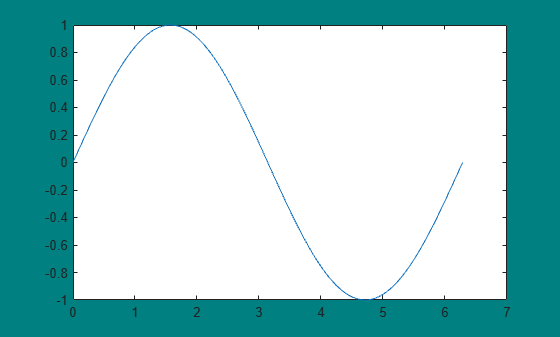clf

Description

example

clf deletes all children of the current figure that have visible handles.

example

clf(fig) deletes all children of the specified figure that have visible handles.

example

clf('reset') deletes all children of the current figure regardless of their handle visibility. It also resets the figure properties to their default values, except for the Position, Units, PaperPosition, and PaperUnits properties. Alternatively, you can reset the figure using the clf reset command without parentheses.

example

clf(fig,'reset') deletes all children of the specified figure and resets its properties.

example

f = clf(___) returns the figure for any of the previous syntaxes.

Examples

collapse all

Create a line plot. Then, set the background color of the current figure.

x = linspace(0,2*pi);
y = sin(x);
plot(x,y)

f = gcf;
f.Color = [0 0.5 0.5];Clear the figure using a call to clf. The function call deletes the plot. However, it does not affect the background color of the figure.

clf

Now, reset the figure properties and return the children of the figure. clf('reset') resets the background color to its default value.

f = clf('reset');
f.Children
ans =
0x0 empty GraphicsPlaceholder array.

Create two figures, each with a line plot. Set the background color of the first figure.

f1 = figure('Color','b');
plot(1:10)f2 = figure;
plot((1:10).^2)Clear the line plot from the first figure without resetting the background color of the figure.

clf(f1)

Now, reset all properties of the first figure.

clf(f1,'reset')

Create a figure with a white background. Then, create a surface plot.

figure('Color','w')
peaks

z =  3*(1-x).^2.*exp(-(x.^2) - (y+1).^2) ...
- 10*(x/5 - x.^3 - y.^5).*exp(-x.^2-y.^2) ...
- 1/3*exp(-(x+1).^2 - y.^2)Clear the surface plot from the figure and reset all figure properties to their default values. clf reset resets all properties of the current figure, except for the Position, Units, PaperPosition, and PaperUnits properties.

clf reset

Input Arguments

collapse all

Target figure, specified as a Figure object or figure number. Use fig to clear a specific figure, instead of the current figure.

If fig is a figure number, MATLAB® searches for an existing figure in which the Number property is equal to fig. By default, the Number property value is displayed in the title of the figure.

Example: clf(f) clears the figure with handle f.

Example: clf(1) clears the figure with number 1.

collapse all

Visible Handles

A handle is visible to clf if the HandleVisibility property of the object is set to 'on'. When you call clf in the Command Window or within a callback routine, the function deletes only those objects whose HandleVisibility property is set to 'on'. It does not delete objects whose HandleVisibility property is set to 'callback' or 'off'.

Tips

• To clear the contents of a figure, you can alternatively use Clear Figure from the figure window's Edit menu. Using Clear Figure deletes all children of the figure that have visible handles.